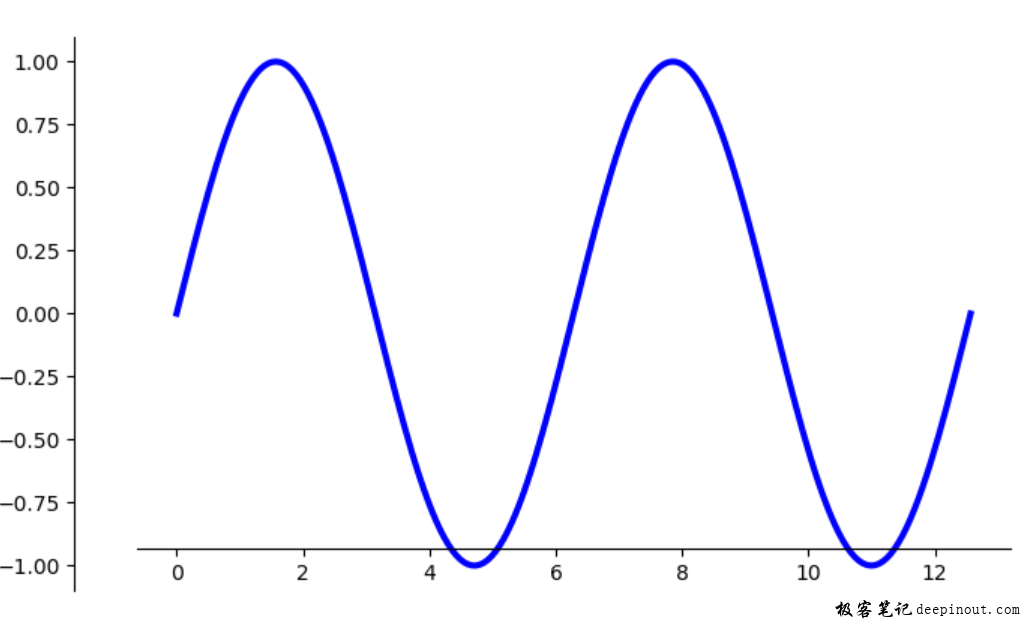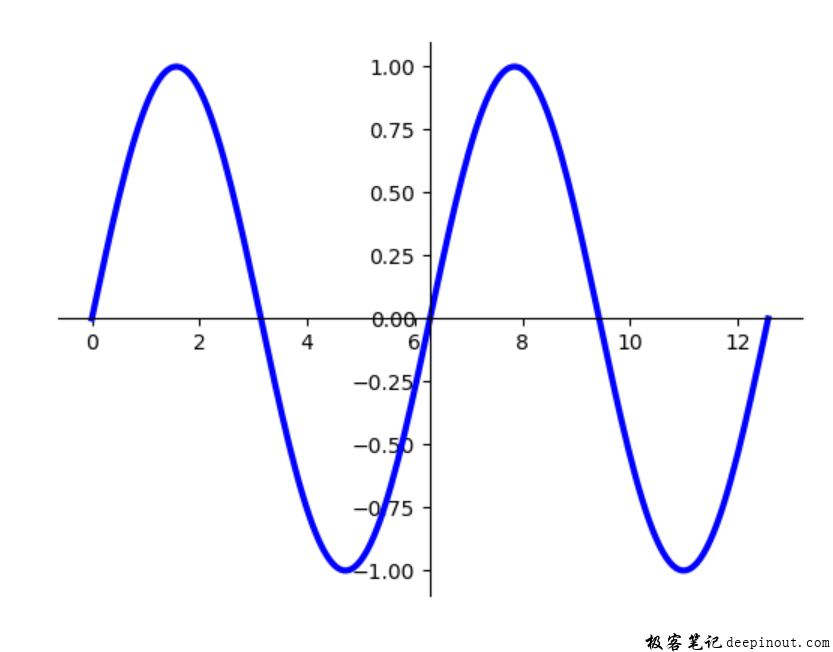# Matplotlib 与数据区图像相对位置移动坐标轴位置

Matplotlib 移动坐标轴位置学习了根据数据在坐标轴上表示的位置来调整坐标轴的位置，这是移动坐标轴的第一种方式，接着下来介绍第二种方式，如果没有看过前文，建议先查看前面的文章，否则看本文就有点云里雾里，总是身在此山中不知在何处。ax.spines['left'].set_position(("outward", 30))
ax.spines['bottom'].set_position(("outward", -20))


import numpy as np
import matplotlib.pyplot as plt
from matplotlib.ticker import FuncFormatter, MaxNLocator

#
fig, ax = plt.subplots()  #创建子图

#
x = np.linspace(0, 4*np.pi, 200)
y = np.sin(x)

#
ax.plot(x, y, lw = 3, color = 'b')

#隐藏右边和上边
ax.spines['right'].set_visible(False)
ax.spines['top'].set_visible(False)

#
ax.spines['left'].set_position(("outward", 30))
ax.spines['bottom'].set_position(("outward", -20))

plt.show()import numpy as np
import matplotlib.pyplot as plt
from matplotlib.ticker import FuncFormatter, MaxNLocator

#
fig, ax = plt.subplots()  #创建子图

#
x = np.linspace(0, 4*np.pi, 200)
y = np.sin(x)

#
ax.plot(x, y, lw = 3, color = 'b')

#隐藏右边和上边
ax.spines['right'].set_visible(False)
ax.spines['top'].set_visible(False)

#
ax.spines['left'].set_position(("axes", 0.5))
ax.spines['bottom'].set_position(("axes", 0.5))

plt.show()


'center' -> ('axes', 0.5)
'zero' -> ('data', 0.0)


• 回顶